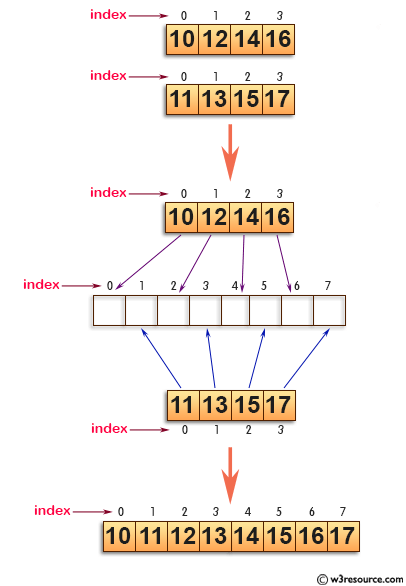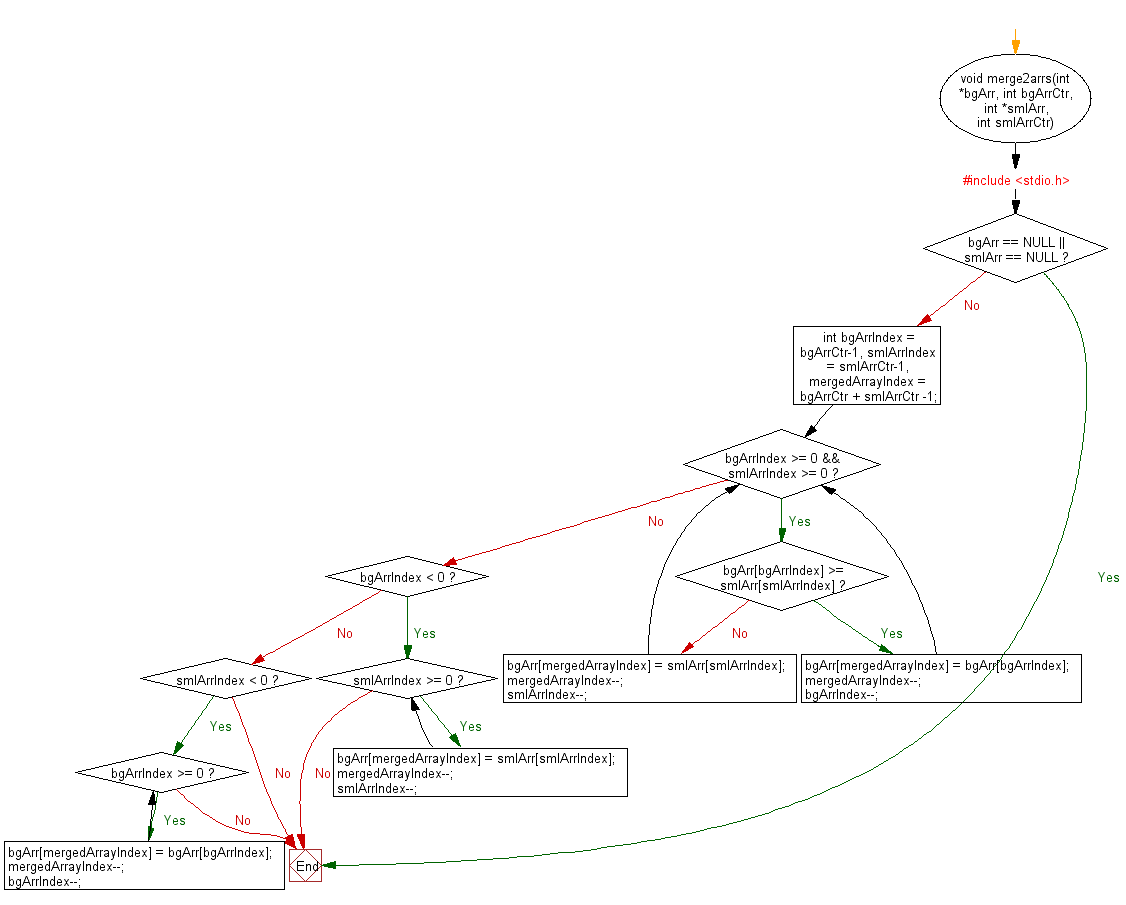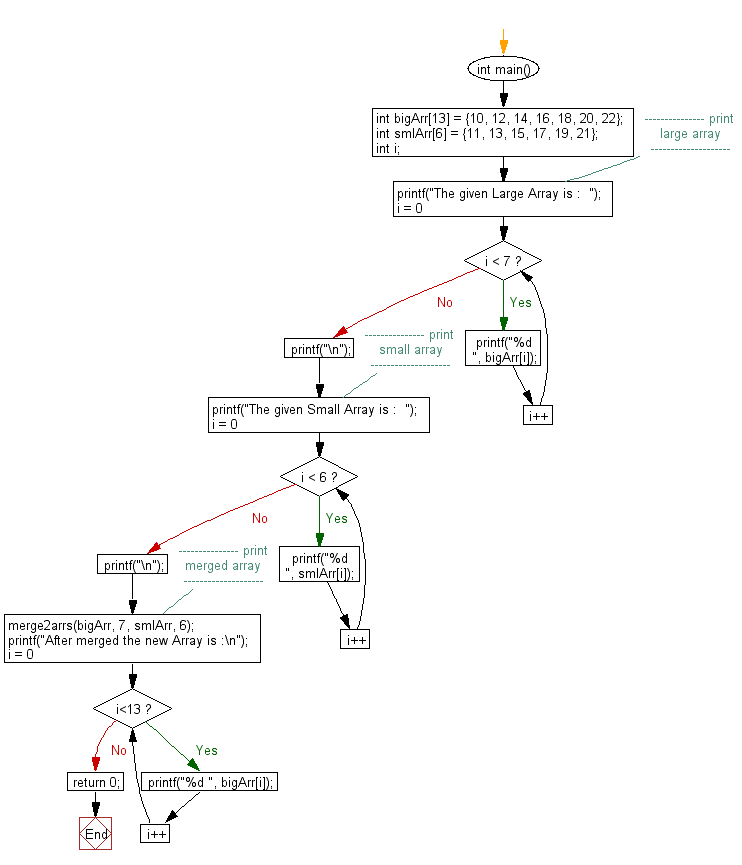﻿ C exercises: Merge one sorted array into another sorted array - w3resource# C Exercises: Merge one sorted array into another sorted array

## C Array: Exercise-38 with Solution

Write a program in C to merge one sorted array into another sorted array.
N.B.: The size of first array is (m+n) but only first m locations are populated remaining are empty. The second array is of size equal to n.

Pictorial Presentation:Sample Solution:

C Code:

``````#include <stdio.h>

void merge2arrs(int *bgArr, int bgArrCtr, int *smlArr, int smlArrCtr)
{
if(bgArr == NULL || smlArr == NULL)
return;
int bgArrIndex = bgArrCtr-1,
smlArrIndex = smlArrCtr-1,
mergedArrayIndex = bgArrCtr + smlArrCtr -1;
while(bgArrIndex >= 0 && smlArrIndex >= 0) {
if(bgArr[bgArrIndex] >= smlArr[smlArrIndex]){
bgArr[mergedArrayIndex] = bgArr[bgArrIndex];
mergedArrayIndex--;
bgArrIndex--;
} else {
bgArr[mergedArrayIndex] = smlArr[smlArrIndex];
mergedArrayIndex--;
smlArrIndex--;
}
}
if(bgArrIndex < 0)
{
while(smlArrIndex >= 0)
{
bgArr[mergedArrayIndex] = smlArr[smlArrIndex];
mergedArrayIndex--;
smlArrIndex--;
}
} else if (smlArrIndex < 0)
{
while(bgArrIndex >= 0)
{
bgArr[mergedArrayIndex] = bgArr[bgArrIndex];
mergedArrayIndex--;
bgArrIndex--;
}
}
}

int main()
{
int bigArr = {10, 12, 14, 16, 18, 20, 22};
int smlArr = {11, 13, 15, 17, 19, 21};
int i;
//--------------- print large array --------------------
printf("The given Large Array is :  ");
for(i = 0; i < 7; i++)
{
printf("%d  ", bigArr[i]);
}
printf("\n");
//--------------- print small array --------------------
printf("The given Small Array is :  ");
for(i = 0; i < 6; i++)
{
printf("%d  ", smlArr[i]);
}
printf("\n");
//--------------- print merged array --------------------
merge2arrs(bigArr, 7, smlArr, 6);
printf("After merged the new Array is :\n");
for(i = 0; i<13; i++)
{
printf("%d ", bigArr[i]);
}
return 0;
}
```
```

Sample Output:

```The given Large Array is :  10  12  14  16  18  20  22
The given Small Array is :  11  13  15  17  19  21
After merged the new Array is :
10 11 12 13 14 15 16 17 18 19 20 21 22
```

Flowchart: 1Flowchart: 2C Programming Code Editor:

Improve this sample solution and post your code through Disqus.

What is the difficulty level of this exercise?

﻿